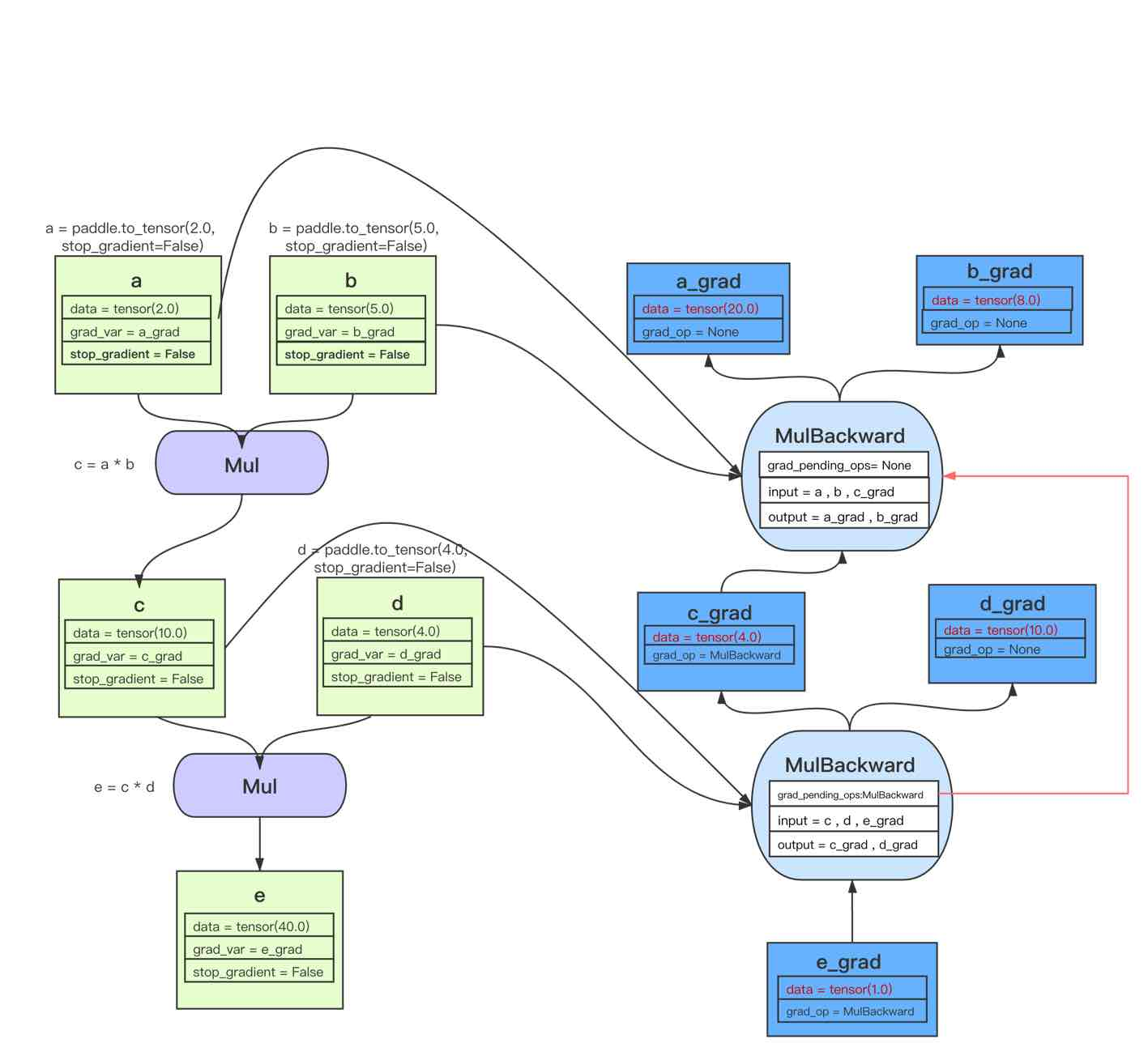# 自动微分机制介绍¶

## 一、背景¶

• 前向传播是输入通过每一层节点计算后得到每层输出，上层输出又作为下一层的输入，最终达到输出层。然后通过损失函数计算得到 loss 值。

• 反向传播是通过 loss 值来指导前向节点中的函数参数如何改变，并更新每层中每个节点的参数，来让整个神经网络达到更小的 loss 值。

## 二、如何使用飞桨的自动微分机制¶

```#加载飞桨和相关类库
import numpy as np

```
```2.2.0
```

```model = vgg11()

```

```# 前向传播
predicts = model(x)
```

```# 计算损失
loss = F.cross_entropy(predicts, label)
```

```# 开始进行反向传播
loss.backward()
```

```# 设置优化器
```

```# 更新参数
optim.step()
```

## 三、飞桨中自动微分相关所有的使用方法说明¶

1、飞桨中的`Tensor``stop_gradient`属性，这个属性可以查看一个`Tensor`是否计算并传播梯度。

• 如果为`True`，则该`Tensor`不会计算梯度，并会阻绝 Autograd 的梯度传播。

• 反之，则会计算梯度并传播梯度。用户自行创建的的`Tensor`，默认`stop_gradient``True`，即默认不计算梯度；模型参数的`stop_gradient`默认都为`False`，即默认计算梯度。

```import paddle

```
```True
False
```
```a.stop_gradient = False
```
```False
```

2、接下来，本文用一个简单的计算图来了解如何调用`backward()`函数。开始从当前`Tensor`开始计算反向的神经网络，传导并计算计算图中`Tensor`的梯度。

```import paddle

z = x ** 2 + 4 * y
```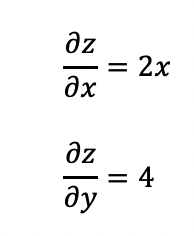```z.backward()
```
```Tensor x's grad is: [2. 4. 6.]
Tensor y's grad is: [4. 4. 4.]
```

```import paddle

y = x + 3
y.backward(retain_graph=True) # 设置 retain_graph 为 True，保留反向计算图
```
```Tensor x's grad is: [1. 1. 1.]
```

3、因为`backward()`会累积梯度，所以飞桨还提供了`clear_grad()`函数来清除当前`Tensor`的梯度。

```import paddle
import numpy as np

x = np.ones([2, 2], np.float32)
inputs2 = []

for _ in range(10):
inputs2.append(tmp)

loss2.backward()

```
```Before clear [1.]
After clear [0.]
```

## 四、飞桨自动微分运行机制¶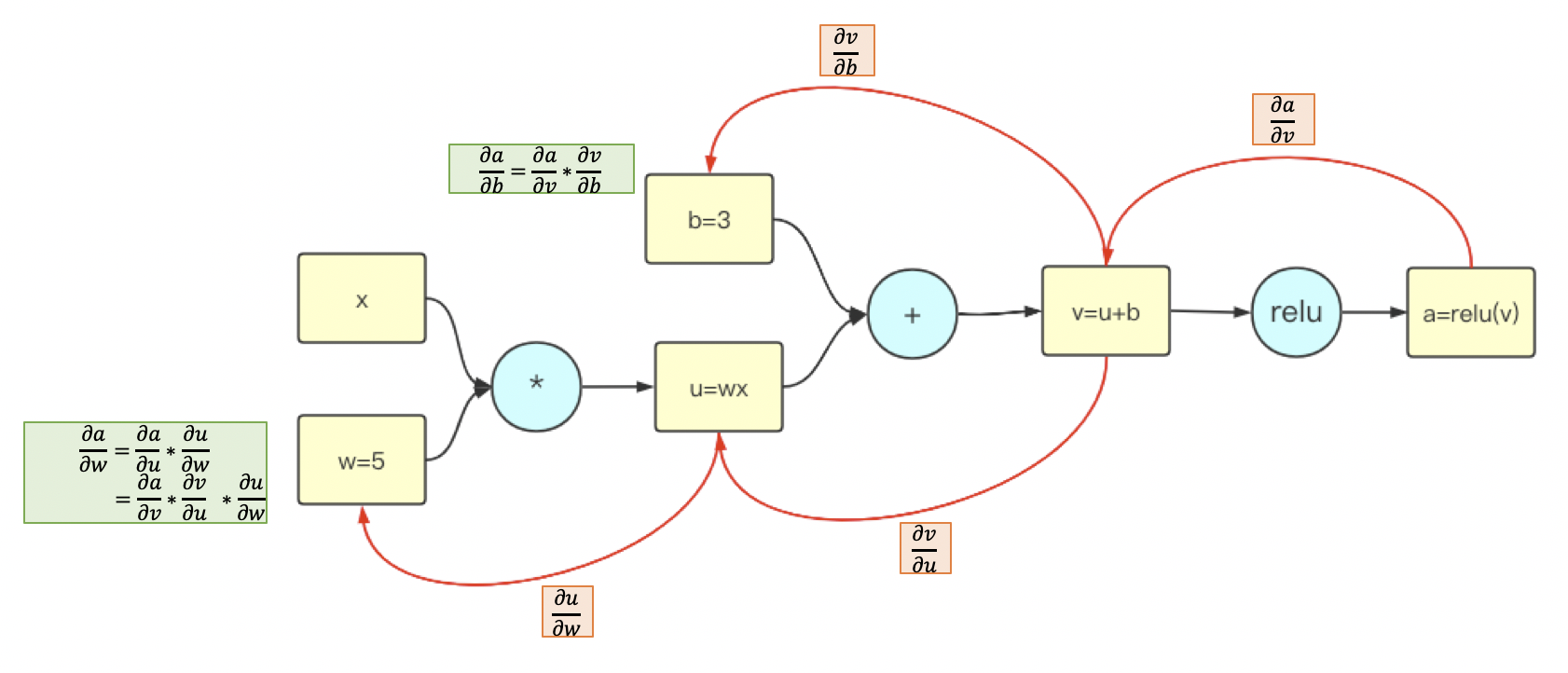```import paddle

c = a * b
c.backward()
```
```Tensor a's grad is: [5.]
```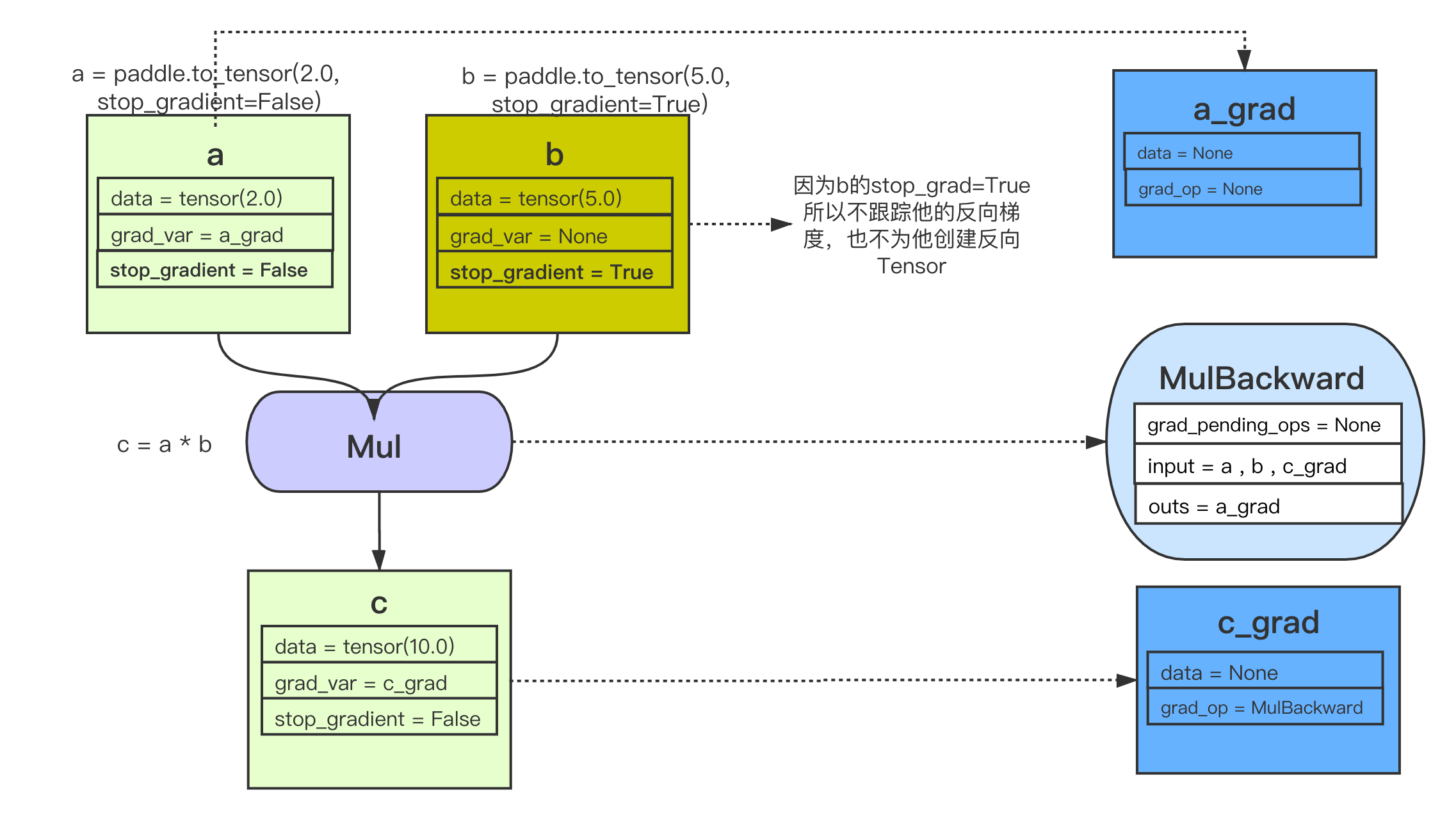-乘法 OP 的反向 OP，即`MulBackward`的输入是，正向 OP 的两个输入，以及正向 OP 的输出 Tensor 的反向 Tensor。在此例子中就是，`a``b``c_grad`

-乘法 OP 的反向 OP，即`MulBackward`的输出是，正向 OP 的两个输入的反向 Tensor（如果输入是 stop_gradient=True，则即为 None）。在此例子中就是，`a_grad``None（b_grad）`

-乘法 OP 的反向 OP，即`MulBackward``grad_pending_ops`是自动构建反向网络的时候，让这个反向 op 知道它下一个可以执行的反向 op 是哪一个，可以理解为反向网络中，一个反向 op 指向下一个反向 op 的边。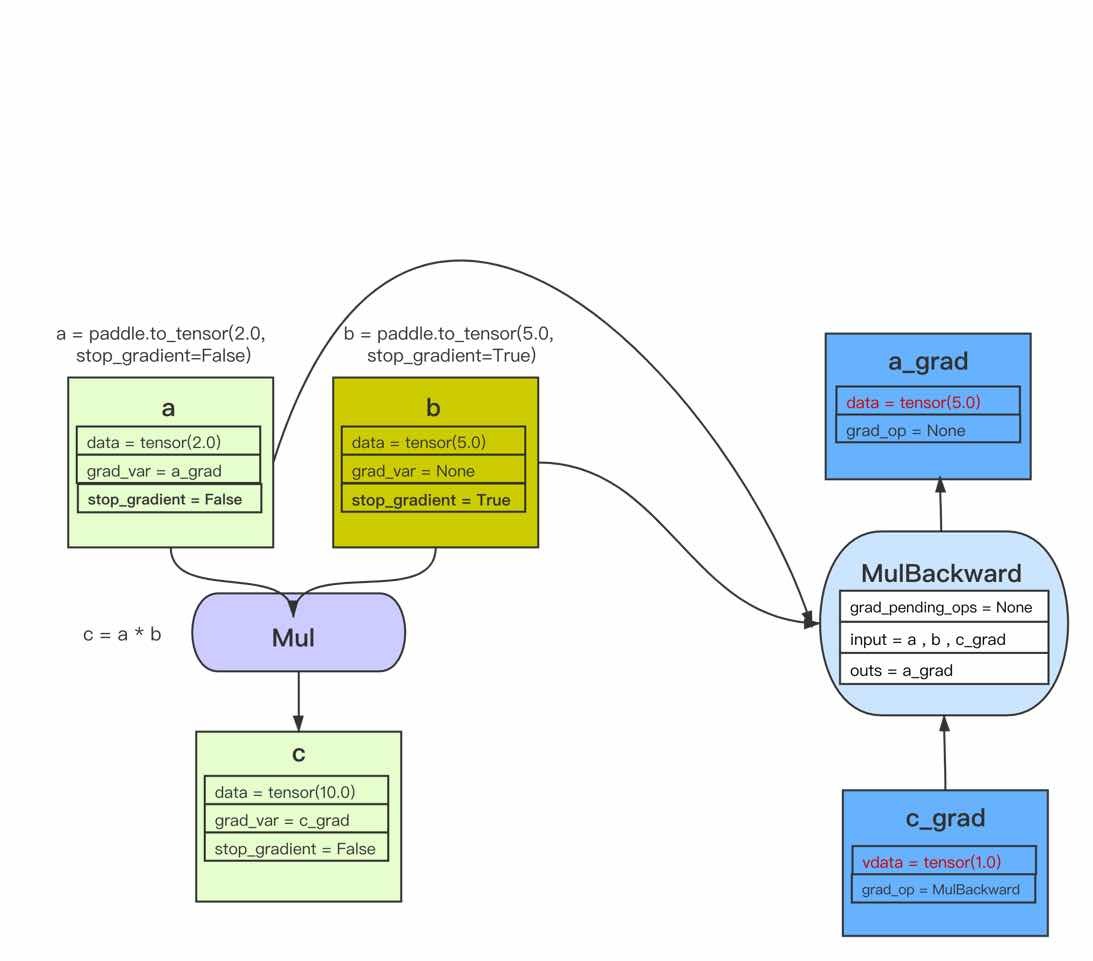```import paddle

c = a * b
e = c * d
e.backward()
```
```Tensor a's grad is: [20.]